# How to display the power of in excel

While working with Excel, you will, in one way or another other, come across the need to raise a number to a power. You can do this easily in Excel without so much hassle. The article illustrates how you can do it. The Excel software has been made to support different ways that you can be able to do this. Here are some of the ways you can be able to display the power in Excel.

## Displaying the power of in excel using the character “^”

This is the easiest and standard option to use when you want to display the power in excel. What you have to do is use the “^” icon. It is on your keyboard, or you can press Shift+6 with the English keyboard layout. Say you want to display 114 to power 8 and 180 to power 2 in cells B5 and C5, respectively. To do this, you will follow the steps:

1. Launch excel and open the worksheet that you want to display value to the power.

2. Select Cell B5, where you want to display 114 to the power of 8 in excel.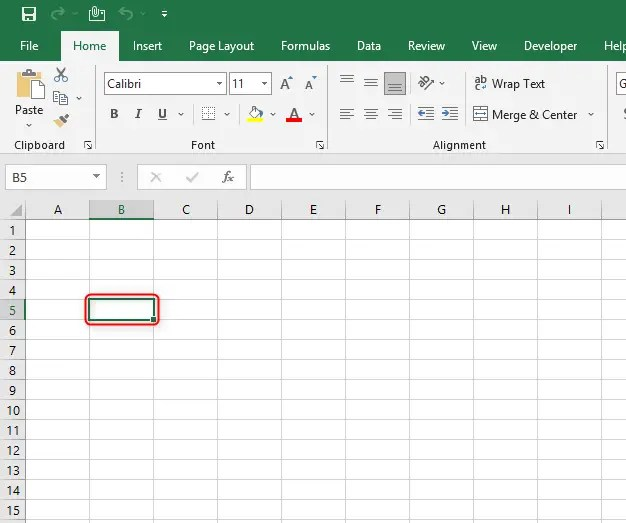3. In the cell type 114^8.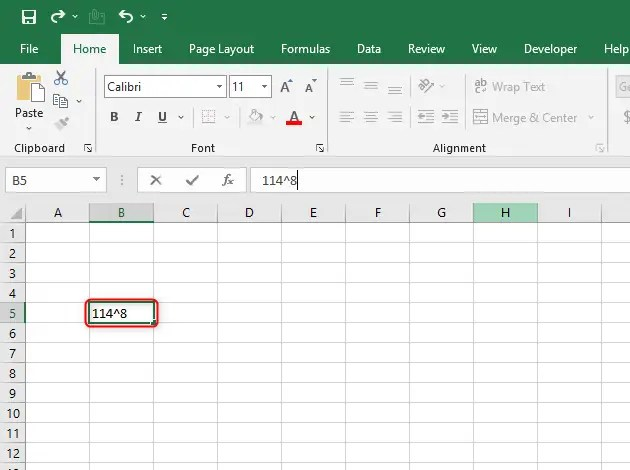4. Then select Enter. You will display 114 to the power of 8 in excel

5. You can calculate the value by introducing the “=” sign just before the numbers.6. Select cell C5. Repeat the procedure, and you will be able to display 180 to the power of 2 in Excel.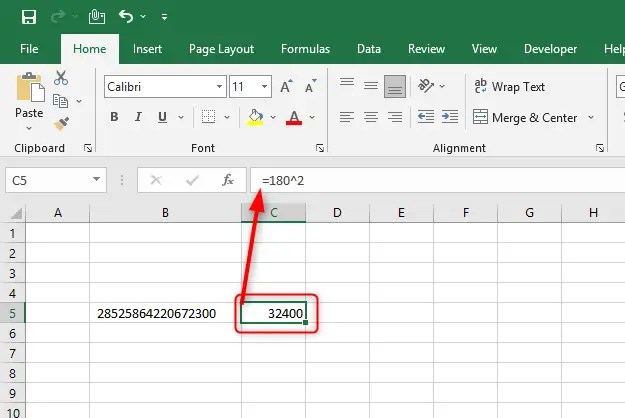Note: The degree(power) is put after the “^” sign. Besides, it is necessary to put the “=” sign in the cell before specifying the power of numbers that you want to display.

## Displaying the power of in excel using the power function formula

The power can also be displayed using the POWER function. Here is the function =POWER(Number, Power)

The formula consists of these two parts Number and Power.

Number: It refers to the basic value which will be used in building the base value of the number to display in the power of. Use real numbers here.

Power: These refer to the value of the degree (index number).

Note: Both parameters can use values that can be less than zero. Just use a “-” sign.

Using our example above in cell B5, type =POWER (114,8), type = POWER (180,2) as well in cell C5.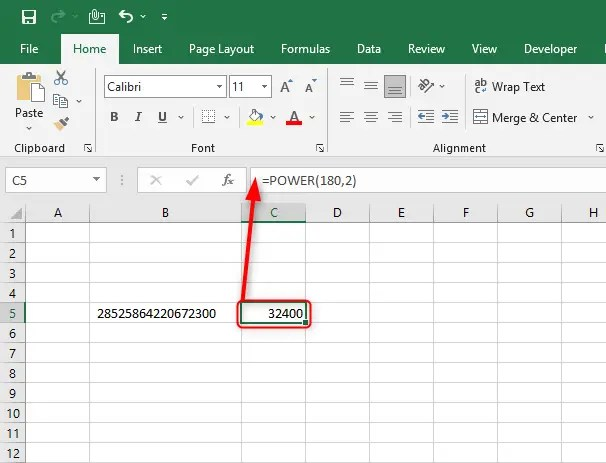## Displaying the power of Excel using the superscript option

Using this option, you can be able to display the number neatly and beautifully on the table for printing. What you have to do is employ the use of the format cell tab. In our example above, we can display the two numbers in cells B5 and C5, respectively. What you have to do is

1. Select cell B5.

2. Type 1148, then now, in the formula bar, select 8.

3. Click on the Home tab of the main menu ribbon.

4. In the Font group, click on the Font setting Arrow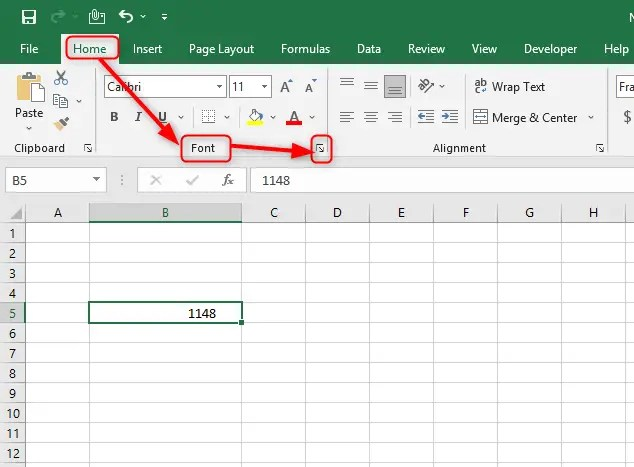5. A pop-screen Format cells menu appears.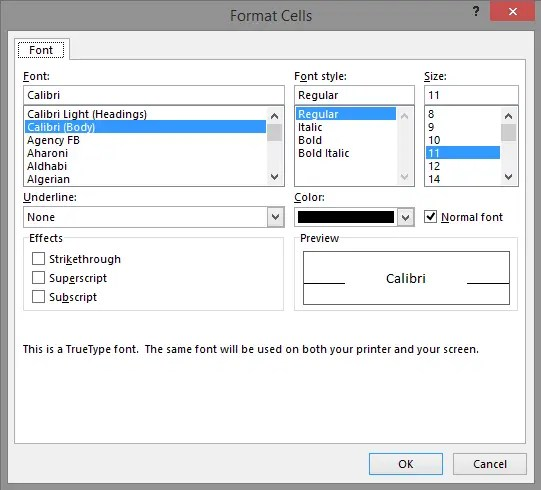6. In the Format cells popup menu, tick the “Superscript” option and click OK.7. There you go. You have displayed the power of Excel. Repeat the process for cell C5.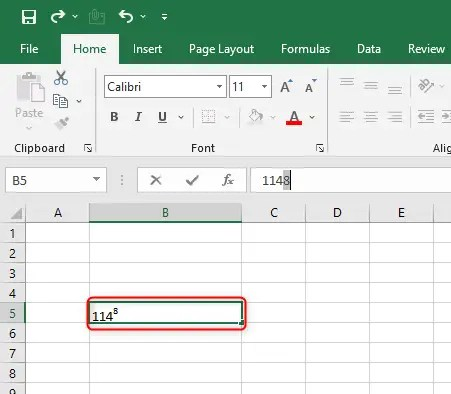## How to type to the power of ½ in Excel?

The other name of superscript is known as the power of in scientific and arithmetic terms. So typing to the power of ½ in Excel is the same as typing ½ as a superscript. This can easily be done in word processing applications such as Microsoft Word and Google Docs. But in Excel and Google Sheets, it’s a little bit complicated.

### By using the superscript (power) shortcut (Type A then ALT+ HFNE)

By default, all Microsoft applications come with shortcuts that can be navigated by pressing ALT then, followed by a series of given letters.

To access the superscript option, you need to hold down ALT then, followed by HFNE.

For instance, we want to write 41/2 follow these steps

1. Type 4 in your preferred cell

2. Then enter the shortcut ALT+HFNE to launch the superscript options3. Press OK to save changes

4. Type in ½ and press enter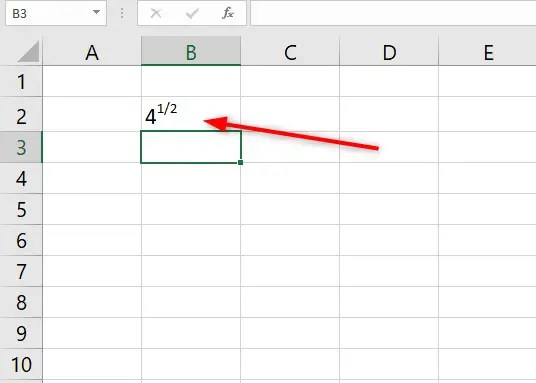### By using the notation ^ symbol

The notation ^ symbol is used to express that the immediate number to the right is a superscript.

So all you need to do is to type the base number then, followed by the notation symbol, and then ½. See the screenshot below for details.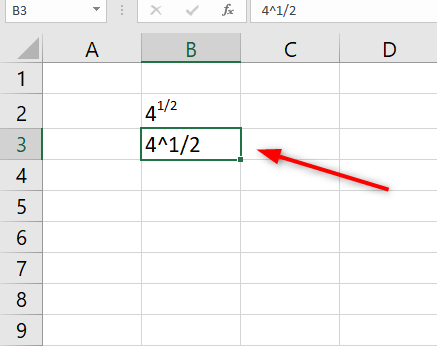## How to type a to the power of 2 in Excel

Typing any letter or number to the power of 2 in excel is easy.

1. Type in any blank cell

2. Type the superscript shortcut ALT + HFNE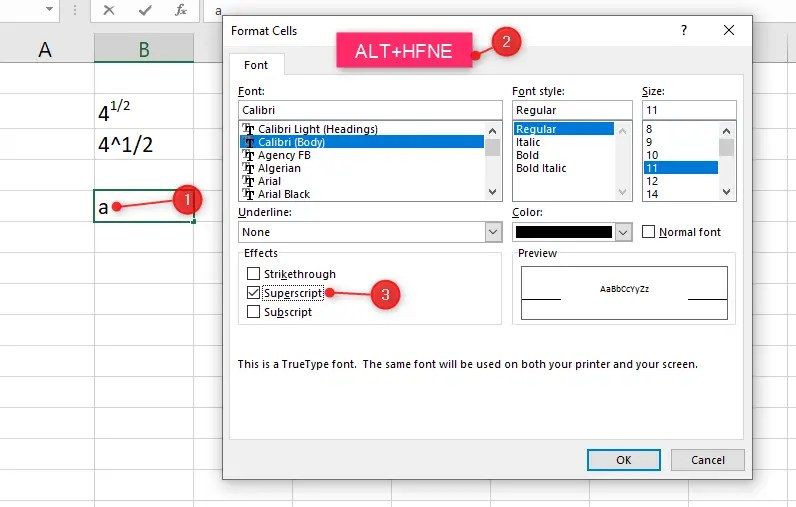3. Press OK

4. Type 2 and press enter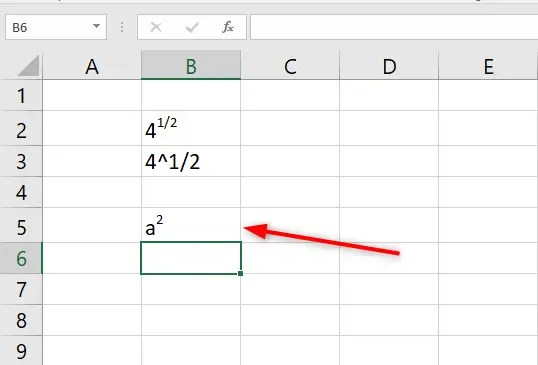Similar Read: How to insert, edit or remove outside border in Excel

## Why is it showing E+ in Excel?

E stands for Exponent. It is a scientific way of showcasing part of a long number by replacing some digits with E+n. In this case, if a number is longer than the size of a cell in Excel, you will get E+n. It is not an error but a way of representing long numbers. You can easily solve this by changing the format of the cell to numbers from the format cells option.

## How to change E+ to numbers in Excel

The easiest way to change E+ to numbers is by enlarging the cell size to hold all numbers. For example, in the screenshot below, cell A1 indicates 4.586E+25All I need to do to convert it into numbers is to change the format of the cell.

1. Right-click on the cell

2. Select the option format cells3. Navigate to the number, select the first option, then press the okay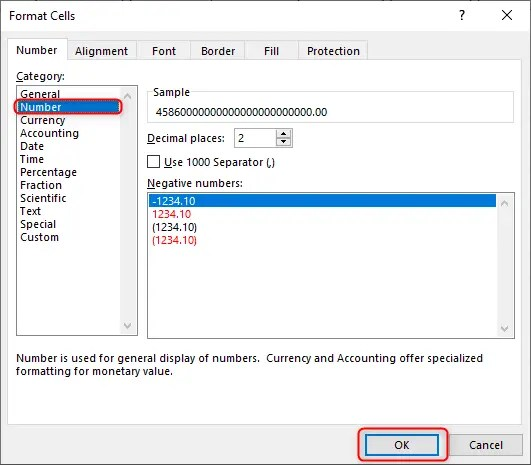4. In case the number is too long, it might display #######. Don’t panic; this is another code representing a number longer than the size of the cell.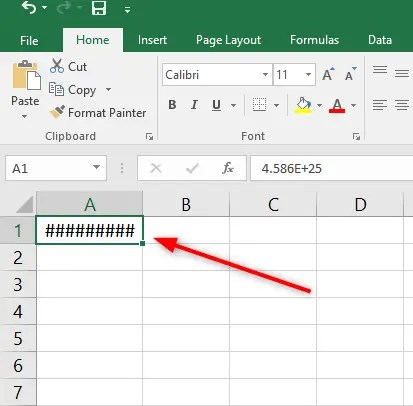5. Just enlarge the cell to display the actual numbers## How to display a subscript in Excel

In Excel, you will come across scenarios that require subscript in many occasions. A subscript can be reffered to as the opposite of a superscript or power notation in Excel. Now that we have seen how to write superscripts let’s see how we can write subscripts.

### By using the format cells option

1. Write the text or string in a blank cell

2. Highlight the portion of the text/string you wish to change into a subscript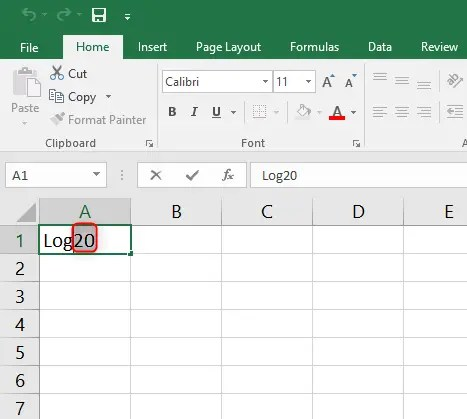3. Place the cursor on the highlighted section and right click then select format cells4. This will launch format cells>> Font. Now tick the subscript option to convert 20 of the log20 text into a subscript.5. Click okay to apply the changes.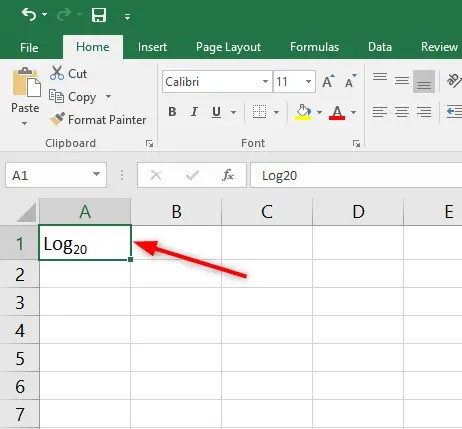As you can see from the above picture, 20 of Log20 has been converted to a subscript. So the new string is Log20.

Superscript practice sheet

### By using the subscript shortcut (ALT+HFNB)

You can insert a subscript in Excel by using the keyboard shortcut, which is ALT+HFNB. For instance, you can write ten as a subscript of Log10 in Excel by following these simple steps

1. Write Log10 in a blank cell

2. Highlight the number 10

3. Hold down Alt and press HFNB. This will launch the format cells options and tick the subscript option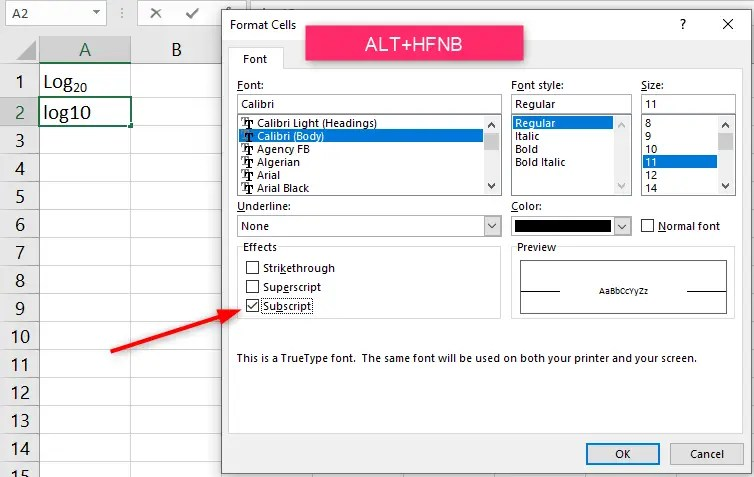4. Click okay to apply changes.As you can see from the image above, Log10 has been converted into Log10. 10 is now a subscript.

### Display subscript by using Ink equation

Excel has a function that allows users to write equations with the help of the mouse. Due to the complexity of equations, subscripts and superscripts are very common. Therefore, you can take advantage of this feature to draw a subscript and any other symbols that you deem appropriate.

Step 1: Select a blank cell

Step 2: Navigate to insert and click on the symbols drop-down, followed by a drop, and lastly, select the ink option.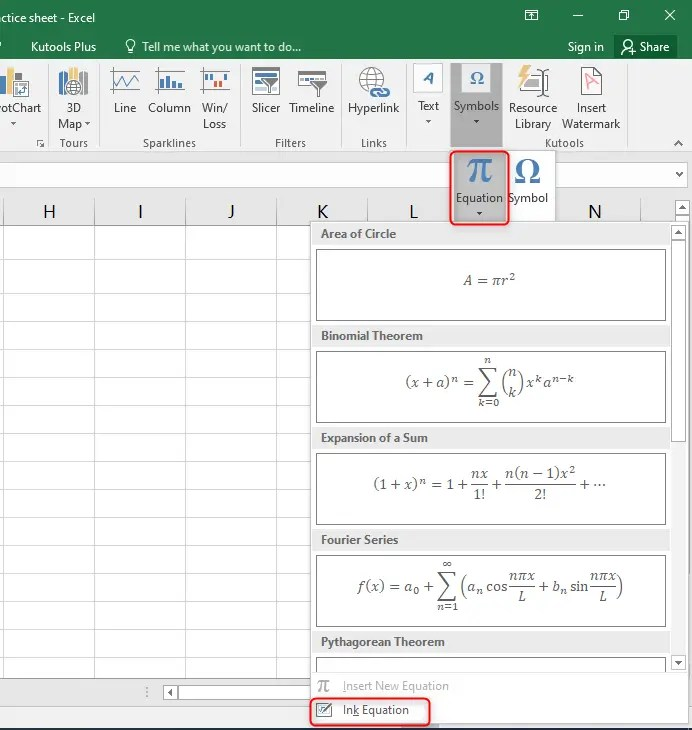Step 3: This will launch a dialog where you can draw the subscript using a mouse. Note that Excel has to identify your handwriting; therefore, it may take several tries.

Tip: Write the subscript as a smaller number right at the foot/base of the previous number or string.Step 4. Once you are done writing the subscript, select insert to place it in an excel cell that you selected earlier.You should note that an ink equation in Excel is inserted in the form of an object. This cannot treat as a valid formula or value.

1. What is the shortcut for superscript in Excel?

The Excel keyboard shortcut for a subscript is ALT+HFNE. Alternatively, you can press CTRL+1 and then select the superscript option.

2. How do you write 15 in Excel?

First, type 15 in a black cell. Highlight five, then hold down ALT and press HFNE. Click okay to apply the changes.

## Final Thoughts

In this article, we have gone through how to display the power of a value in Excel. We have used the same skills to display subscripts since it’s just the opposite of superscripts. Superscript is used to show power notation.

Excel Weez is a free Library for Excel tutorials. Learn excel skills and apply them at school, at the job place, or to solve your daily challenges.